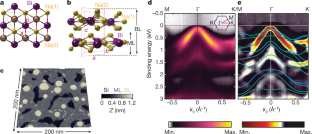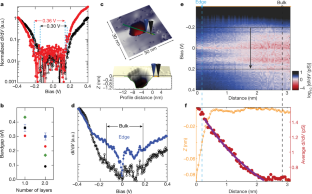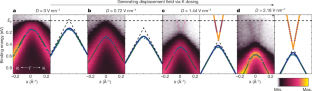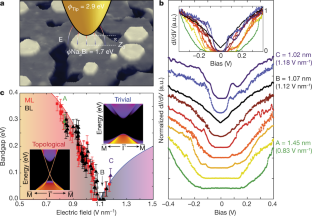# Electric-field-tuned topological phase transition in ultrathin Na3Bi

## Abstract

The electric-field-induced quantum phase transition from topological to conventional insulator has been proposed as the basis of a topological field effect transistor1,2,3,4. In this scheme, ‘on’ is the ballistic flow of charge and spin along dissipationless edges of a two-dimensional quantum spin Hall insulator5,6,7,8,9, and ‘off’ is produced by applying an electric field that converts the exotic insulator to a conventional insulator with no conductive channels. Such a topological transistor is promising for low-energy logic circuits4, which would necessitate electric-field-switched materials with conventional and topological bandgaps much greater than the thermal energy at room temperature, substantially greater than proposed so far6,7,8. Topological Dirac semimetals are promising systems in which to look for topological field-effect switching, as they lie at the boundary between conventional and topological phases3,10,11,12,13,14,15,16. Here we use scanning tunnelling microscopy and spectroscopy and angle-resolved photoelectron spectroscopy to show that mono- and bilayer films of the topological Dirac semimetal3,17 Na3Bi are two-dimensional topological insulators with bulk bandgaps greater than 300 millielectronvolts owing to quantum confinement in the absence of electric field. On application of electric field by doping with potassium or by close approach of the scanning tunnelling microscope tip, the Stark effect completely closes the bandgap and re-opens it as a conventional gap of 90 millielectronvolts. The large bandgaps in both the conventional and quantum spin Hall phases, much greater than the thermal energy at room temperature (25 millielectronvolts), suggest that ultrathin Na3Bi is suitable for room-temperature topological transistor operation.

## Access options

from\$8.99

All prices are NET prices.Fig. 1: Characterization of epitaxial few-layer Na3Bi and overall electronic structure from ARPES.Fig. 2: Bandgap in ML and BL Na3Bi and edge state behaviour.Fig. 3: Band-structure modulation in ARPES in ML and BL Na3Bi using potassium (K) dosing.Fig. 4: Electric-field-induced bandgap modulation of ML and BL Na3Bi in STM.

## Data availability

The data that support the findings of this study are available from the corresponding author upon reasonable request.

## References

1. 1.

Qian, X., Liu, J., Fu, L. & Li, J. Quantum spin Hall effect in two-dimensional transition metal dichalcogenides. Science 346, 1344–1347 (2014).

2. 2.

Liu, J. et al. Spin-filtered edge states with an electrically tunable gap in a two-dimensional topological crystalline insulator. Nat. Mater. 13, 178–183 (2014).

3. 3.

Pan, H., Wu, M., Liu, Y. & Yang, S. A. Electric control of topological phase transitions in Dirac semimetal thin films. Sci. Rep. 5, 14639 (2015).

4. 4.

Vandenberghe, W. G. & Fischetti, M. V. Imperfect two-dimensional topological insulator field-effect transistors. Nat. Commun. 8, 14184 (2017).

5. 5.

König, M. et al. Quantum spin Hall insulator state in HgTe quantum wells. Science 318, 766–770 (2007).

6. 6.

Reis, F. et al. Bismuthene on a SiC substrate: a candidate for a high-temperature quantum spin Hall material. Science 357, 287–290 (2017).

7. 7.

Tang, S. et al. Quantum spin Hall state in monolayer 1T′-WTe2. Nat. Phys. 13, 683–687 (2017).

8. 8.

Fei, Z. et al. Edge conduction in monolayer WTe2. Nat. Phys. 13, 677–682 (2017).

9. 9.

Wu, S. et al. Observation of the quantum spin Hall effect up to 100 kelvin in a monolayer crystal. Science 359, 76–79 (2018).

10. 10.

Liu, Z. K. et al. Discovery of a three-dimensional topological Dirac semimetal, Na3Bi. Science 343, 864–867 (2014).

11. 11.

Wang, Z. et al. Dirac semimetal and topological phase transitions in A3Bi (A=Na, K, Rb). Phys. Rev. B 85, 195320 (2012).

12. 12.

Liu, Z. K. et al. A stable three-dimensional topological Dirac semimetal Cd3As2. Nat. Mater. 13, 677–681 (2014).

13. 13.

Borisenko, S. et al. Experimental realization of a three-dimensional Dirac semimetal. Phys. Rev. Lett. 113, 027603 (2014).

14. 14.

Hellerstedt, J. et al. Electronic properties of high-quality epitaxial topological Dirac semimetal thin films. Nano Lett. 16, 3210–3214 (2016).

15. 15.

Zhang, Y. et al. Molecular beam epitaxial growth of a three-dimensional topological Dirac semimetal Na3Bi. Appl. Phys. Lett. 105, 031901 (2014).

16. 16.

Schumann, T. et al. Observation of the quantum Hall effect in confined films of the three-dimensional Dirac semimetal Cd3As2. Phys. Rev. Lett. 120, 016801 (2018).

17. 17.

Niu, C. et al. Robust dual topological character with spin-valley polarization in a monolayer of the Dirac semimetal Na3Bi. Phys. Rev. B 95, 075404 (2017).

18. 18.

Wu, J. et al. High electron mobility and quantum oscillations in non-encapsulated ultrathin semiconducting Bi2O2Se. Nat. Nanotechnol. 12, 530–534 (2017).

19. 19.

Edmonds, M. T. et al. Spatial charge inhomogeneity and defect states in topological Dirac semimetal thin films of Na3Bi. Sci. Adv. 3, eaao6661 (2017).

20. 20.

Perdew, J. P. et al. Understanding band gaps of solids in generalized Kohn-Sham theory. Proc. Natl Acad. Sci. USA 114, 2801–2806 (2017).

21. 21.

Voit, J. One-dimensional Fermi liquids. Rep. Prog. Phys. 58, 977–1116 (1995).

22. 22.

Ohta, T., Bostwick, A., Seyller, T., Horn, K. & Rotenberg, E. Controlling the electronic structure of bilayer graphene. Science 313, 951–954 (2006).

23. 23.

Zhang, Y. et al. Direct observation of a widely tunable bandgap in bilayer graphene. Nature 459, 820–823 (2009).

24. 24.

Deng, B. et al. Efficient electrical control of thin-film black phosphorus bandgap. Nat. Commun. 8, 14474 (2017).

25. 25.

Ju, L. et al. Tunable excitons in bilayer graphene. Science 358, 907–910 (2017).

26. 26.

Blöchl, P. E. Projector augmented-wave method. Phys. Rev. B 50, 17953–17979 (1994).

27. 27.

Perdew, J. P., Burke, K. & Ernzerhof, M. Generalized gradient approximation made simple. Phys. Rev. Lett. 77, 3865–3868 (1996).

28. 28.

Souza, I., Marzari, N. & Vanderbilt, D. Maximally localized Wannier functions for entangled energy bands. Phys. Rev. B 65, 035109 (2001).

29. 29.

Lopez Sancho, M. P., Lopez Sancho, J. M., Sancho, J. M. L. & Rubio, J. Highly convergent schemes for the calculation of bulk and surface Green functions. J. Phys. F 15, 851–858 (1985).

30. 30.

Wu, Q., Zhang, S., Song, H. F., Troyer, M. & Soluyanov, A. A. WannierTools: An open-source software package for novel topological materials. Comput. Phys. Commun. 224, 405–416 (2018).

31. 31.

Becke, A. D. & Johnson, E. R. A simple effective potential for exchange. J. Chem. Phys. 124, 221101 (2006).

32. 32.

Tran, F. & Blaha, P. Accurate band gaps of semiconductors and insulators with a semilocal exchange-correlation potential. Phys. Rev. Lett. 102, 226401 (2009).

33. 33.

Koller, D., Tran, F. & Blaha, P. Improving the modified Becke-Johnson exchange potential. Phys. Rev. B 85, 155109 (2012).

34. 34.

Edmonds, M. T., Hellerstedt, J., O’Donnell, K. M., Tadich, A. & Fuhrer, M. S. Molecular doping the topological Dirac semimetal Na3Bi across the charge neutrality point with F4-TCNQ. ACS Appl. Mater. Interfaces 8, 16412–16418 (2016).

35. 35.

Damascelli, A., Hussain, Z. & Shen, Z.-X. Angle-resolved photoemission studies of the cuprate superconductors. Rev. Mod. Phys. 75, 473–541 (2003).

36. 36.

Hufner, S. Photoelectron Spectroscopy: Principles and Applications 3rd edn (Springer, Berlin, 2003)

37. 37.

Battisti, I. et al. Poor electronic screening in lightly doped Mott insulators observed with scanning tunneling microscopy. Phys. Rev. B 95, 235141 (2017).

38. 38.

Yu, R., Qi, X. L., Bernevig, A., Fang, Z. & Dai, X. Equivalent expression of Z2 topological invariant for band insulators using the non-abelian Berry connection. Phys. Rev. B 84, 075119 (2011).

39. 39.

Soluyanov, A. A. & Vanderbilt, D. Wannier representation of Z2 topological insulators. Phys. Rev. B 83, 035108 (2011).

40. 40.

Lang, N. D. Apparent barrier height in scanning tunnelling microscopy. Phys. Rev. B 37, 10395–10398 (1988).

41. 41.

Blanco, J. M., Flores, F. & Perez, R. STM-theory: image potential, chemistry and surface relaxation. Prog. Surf. Sci. 81, 403–443 (2006).

42. 42.

Pitarke, J. M., Echenique, P. M. & Flores, F. Apparent barrier height for tunneling electrons in STM. Surf. Sci. 217, 267–275 (1989).

## Acknowledgements

M.T.E. was supported by ARC DECRA fellowship DE160101157. M.T.E., J.L.C., C.L. and M.S.F. acknowledge funding support from CE170100039. J.L.C., J.H. and M.S.F. are supported by M.S.F.’s ARC Laureate Fellowship (FL120100038). S.A. acknowledges funding support from ARC Discovery Project DP150103837. M.T.E. and A.T. acknowledge travel funding provided by the International Synchrotron Access Program (ISAP) managed by the Australian Synchrotron, part of ANSTO, and funded by the Australian Government. M.T.E. acknowledges funding from the Monash Centre for Atomically Thin Materials Research and Equipment Scheme. S.A.Y. and W.W. acknowledge funding from Singapore MOE AcRF Tier 2 (grant no. MOE2015-T2-2-144). S.A. acknowledges the National University of Singapore Young Investigator Award (R-607-000-094-133). This research used resources of the Advanced Light Source, which is a DOE Office of Science User Facility under contract no. DE-AC02-05CH11231. Part of this research was undertaken on the soft X-ray beamline at the Australian Synchrotron, part of ANSTO. The authors acknowledge computational support from the National Supercomputing Centre, Singapore.

## Author information

Authors

### Contributions

M.T.E., J.L.C. and M.S.F. devised the STM experiments. M.T.E. devised the ARPES and XPS experiments. M.T.E. and J.L.C. performed the MBE growth and STM/STS measurements at Monash University. J.H. assisted with the experimental setup at Monash University. M.T.E., J.L.C. and A.T. performed the MBE growth and ARPES measurements at Advanced Light Source with the support from H.R., S.T. and S.-K.M. The MBE growth and XPS measurements at the Australian Synchrotron were performed by M.T.E., J.L.C., A.T., J.H. and C.L. The DFT calculations were performed by L.C.G., J.N.B.R., W.W. and S.A.Y.; S.A. assisted with the theoretical interpretation of the data. M.T.E., J.L.C. and M.S.F. composed the manuscript. All authors read and contributed feedback to the manuscript.

### Corresponding author

Correspondence to Mark T. Edmonds.

## Ethics declarations

### Competing interests

The authors declare no competing interests.

Publisher’s note: Springer Nature remains neutral with regard to jurisdictional claims in published maps and institutional affiliations.

## Extended data figures and tables

### Extended Data Fig. 1 RHEED, LEED and XPS characterization of few-layer Na3Bi.

a, b, RHEED patterns of Si(111) 7 × 7 reconstruction along the $$\bar{{\rm{\Gamma }}}-\bar{{\rm{M}}}$$ direction (a) and of few-layer Na3Bi along the $$\bar{{\rm{\Gamma }}}-\bar{{\rm{K}}}$$ direction (b). c, 1 × 1 LEED image of few-layer Na3Bi taken at 32 eV. d, XPS of Na 2p and Bi 5d core level taken at hv = 100 eV for few-layer Na3Bi. e, Normalized XPS of Si 2p core level taken at 350 eV (left panel) and at 850 eV (right panel). Each panel shows the Si 2p of the clean Si substrate (black curve) and with few-layer Na3Bi grown on top (red curve). The spectra have been offset in intensity for clarity.

### Extended Data Fig. 2 kz band dispersion of few-layer Na3Bi from photon-energy-dependent ARPES measurements.

These photon-energy-dependent ARPES measurements of ML/BL Na3Bi demonstrate effectively 2D dispersion. The figure shows a cut through the photon-energy-dependent (hv = 45–55 eV) Fermi surface, demonstrating non-dispersion of the gapped Dirac valence band along kz, with the intensity of emitted photoelectrons reflected in the colour scale.

### Extended Data Fig. 3 Bandgap extraction from scanning tunnelling spectra.

a, b, dI/dV spectra taken for ML (red) and BL (black) Na3Bi plotted on a linear (a) and a logarithmic (b) scale. The logarithmic scale better accounts for the large change in intensity near the band edge. c, Normalized dI/dV spectra at various tip–sample separations, illustrating that the onset in intensity typically occurs at a normalized dI/dV signal of 0.01 within an error of ±25 meV (when also accounting for tip-induced band bending). d, Calculated tip-induced band bending, ϕBB, using equation (3) for different biases.

### Extended Data Fig. 4 DFT calculations on the topological nature of Na3Bi layers.

a, 2D Brillouin zone for Na3Bi layered structures. Here we also show the projected 1D Brillouin zone used for studying the edge spectrum. bh, Results for ML (bf) and BL (gk) Na3Bi with Na(2) vacancies (with one Na(2) vacancy in a 2 × 2 supercell). b, g, Electronic band structures, where the energy zero is set to be at the valence band maximum. c, h, Orbitally resolved band structure without SOC and d, i, orbitally resolved band structure with SOC. The red dots represent the contribution from the Na s and Bi s atomic orbitals, and the blue dots represent contribution from the Bi px/py atomic orbitals. Band inversion induced by SOC can be clearly observed at the Γ point for both ML and BL cases, which indicates that both ML and BL Na3Bi are non-trivial 2D topological insulators. e, j, Projected edge spectrum (edge along the  direction), where pairs of $${{\mathbb{Z}}}_{2}$$ topological edge states can be observed in the energy gaps (marked by the white arrows) where the colour scale reflects the spectral weight. f, k, Calculated Wannier function centre evolutions, which indicate a nontrivial $${{\mathbb{Z}}}_{2}$$ invariant ($${{\mathbb{Z}}}_{2}=1$$) for the bulk band structure in both ML and BL.

### Extended Data Fig. 5 Extracting and fitting the dispersion relation from ARPES MDC and EDC spectra of few-layer Na3Bi.

a, ARPES intensity plot (colour scale at right) along the K–Γ–K direction after 30 min of K dosing. b, Stack plots of MDCs for the valence band (left panel) and conduction band (right panel) extracted from a, where the blue circles represent the Gaussian-fit band locations. Red and yellow lines mark shared constant-energy kx vertices used throughout b and c. c, EDCs extracted from a. d, e, Fitting band coordinates that have been extracted by MDC and EDC analysis (red dots) to a hyperbola (d) and to a parabola (e), showing that Na3Bi-like bands are best described by a hyperbola function.

### Extended Data Fig. 6 Displacement field calculations from K dosing in ARPES.

a, Electron-band Fermi surface of few-layer Na3Bi after 30 min of K dosing, where the colour scale reflects the intensity of emitted photoelectrons at the Fermi energy. b, Red line, radially averaged momentum profile through the Fermi surface, showing the ring structure at kF (dashed blue line shows position of kF). c, Calculated charge density (left-hand y axis) using equation (7) and the corresponding electric displacement field (right-hand y axis) associated with the net charge transfer from the as-grown film as a function of K-dosing time. Red points are as-measured, and green points are extrapolated based on the EF shifting rate with K dosing between 15 min and 50 min.

### Extended Data Fig. 7 Electric displacement field-dependence of topological insulator ML/BL Na3Bi bands near EF.

a, The sum of Δ parameters from the best fit of equation (5) to ARPES dispersion versus applied electric displacement field. Both Δn,p are directly calculated from the high-field (purple) measurements, however in low-field (green) measurements, EF is not sufficiently shifted for the electron dispersion to be clearly resolved. Here we have used the ratio (Δp + Δn)/Δp ≈ 1.4 measured from the purple points to extrapolate Δn for undosed film. b, The valence edge (blue) and conduction edge (red) calculated from the fitted ARPES dispersion are shown for two high-symmetry directions: circles for K–Γ–K and squares for M–Γ–M.

### Extended Data Fig. 8 Electric-field calculations from STM.

a, b, Tunnelling current as a function of relative tip–sample distance z for a, Au(111) (bias +500 mV), and b, thin film Na3Bi (bias −300 mV). The top axis in b represents the total distance, s, between tip and sample determined as described in Methods section ‘Calculating tip–sample separation and electric field’. The black lines in a and b are exponential fits. c, Energy-level schematic illustrating the effects of an image potential on the (purple trapezoidal) junction barrier, where s is the tip–sample distance, and ϕSample and ϕTip are the work function of the sample and tip, respectively. Progression from blue, green, yellow, orange and red curves indicates modification of the apparent barrier height due to the imaging potential at decreasing tip–sample distances s. d, ln(I(s)/I0) shown as a function of distance for a square barrier model (blue dashed line), for DFT-LDA (black full line), for the image potential model (black dashed-dotted), and for the local orbital basis model (red line). Data are taken from figure 10 of ref. 41.

### Extended Data Fig. 9 Scanning tunnelling spectra of ML Na3Bi.

Shown are individual dI/dV spectra taken on ML Na3Bi at different tip–sample separations (electric field) (shown at right). The spectra have been normalized and offset for clarity.

### Extended Data Fig. 10 DFT calculations of Na3Bi layers with electric field.

a, Calculated bandgap variation as a function of electric field for ML Na3Bi with an Na(2) vacancy. The gap closes and reopens at about 1.85 V Å−1. b, 2D Brillouin zone and the projected 1D boundary Brillouin zone. cf, Orbital-resolved band structures and edge spectra for ML Na3Bi at electric fields of 0.0 V Å−1 (c, d) and 2.5 V Å−1 (e, f), which are marked by points A and B in a. c, Orbital-resolved band structures with SOC in the absence of electric field (the energy zero is set to be at the valence band maximum at the Γ point). The red dots represent the contribution from the Na s and Bi s atomic orbitals, and the blue dots represent the contribution from the Bi px/py atomic orbitals. A band inversion is observed at the Γ point induced by SOC, indicating that ML Na3Bi is a non-trivial 2D topological insulator. d, Projected edge spectrum (edge along the  direction) in the absence of electric field, showing topological edge states. Panels e and f show the corresponding results at an electric field of 2.5 V Å−1. In e, the band ordering at Γ is inverted compared to c, indicating a topological phase transition to a trivial insulator phase. This is confirmed by the disappearance of topological edge states as shown in f.

### Extended Data Fig. 11 Crystal structure and symmetries of ML and BL Na3Bi.

a, b, Tables showing the point group (PG) symmetries for ML and BL Na3Bi, respectively. The second and third rows of each table indicate the effects of electric field (Ez) and an Na(2) vacancy, respectively. We note that at zero electric field both pristine and Na(2) vacancy ML and BL Na3Bi are 2D QSH insulators, with topological index $$\upsilon \in {{\mathbb{Z}}}_{2}=1$$. ch, Crystal structure of Na3Bi as a result of an Na(2) vacancy and electric field. c, f, Pristine ML (c) and BL (f) Na3Bi; d, g, ML (d) and BL (g) Na3Bi each with an Na surface vacancy; e, h, ML (e) and BL (h) Na3Bi with Na surface vacancy plus electric field applied perpendicular to the sample.

## Rights and permissions

Reprints and Permissions

• ### Fe dopants and surface adatoms versus nontrivial topology of single-crystalline Bi 2 Se 3

• M Chrobak
• , K Maćkosz
• , M Jurczyszyn
• , M Dobrzański
• , K Nowak
• , T Ślęzak
• , M Zając
• , M Sikora
• , M Rams
• , T Eelbo
• , J Stępień
• , M Waśniowska
• , O Mathon
• , F Yakhou-Harris
• , D G Merkel
• , I Miotkowski
• , Z Kąkol
• , A Kozłowski
• , M Przybylski
•  & Z Tarnawski

New Journal of Physics (2020)

• ### Normal-to-topological insulator martensitic phase transition in group-IV monochalcogenides driven by light

• Jian Zhou
• , Shunhong Zhang
•  & Ju Li

NPG Asia Materials (2020)

• ### Dual topological insulator device with disorder robustness

• Bruno Focassio
• , Gabriel R. Schleder
• , Armando Pezo
• , Marcio Costa

Physical Review B (2020)

• ### Transport in two-dimensional topological materials: recent developments in experiment and theory

• Dimitrie Culcer
• , Aydın Cem Keser
• , Yongqing Li
•  & Grigory Tkachov

2D Materials (2020)

• ### Importance of interactions for the band structure of the topological Dirac semimetal Na3Bi

• I. Di Bernardo
• , J. Collins
• , W. Wu
• , Ju Zhou
• , Shengyuan A. Yang
• , Sheng Ju
• , M. T. Edmonds
•  & M. S. Fuhrer

Physical Review B (2020)